# 10. DETAILS ZILLDIFFEQMODAP11 7.2.041. MY NOTES ASK YOUR TEACHER Use the Laplace transform to solve the...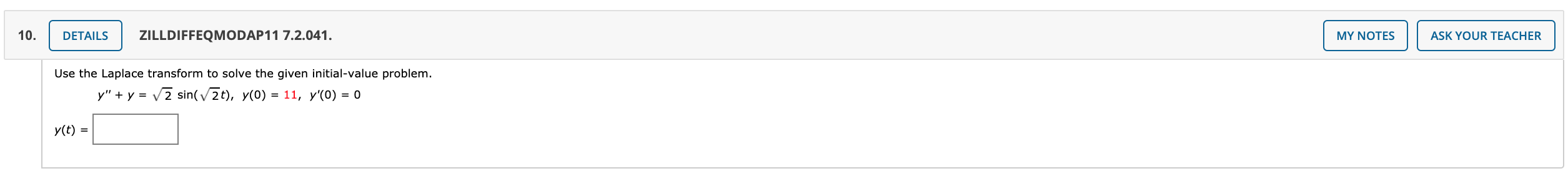10. DETAILS ZILLDIFFEQMODAP11 7.2.041. MY NOTES ASK YOUR TEACHER Use the Laplace transform to solve the given initial-value problem. Y" + y = V2 sin(2t), y(0) = 11, y'(O) = 0 y(t) =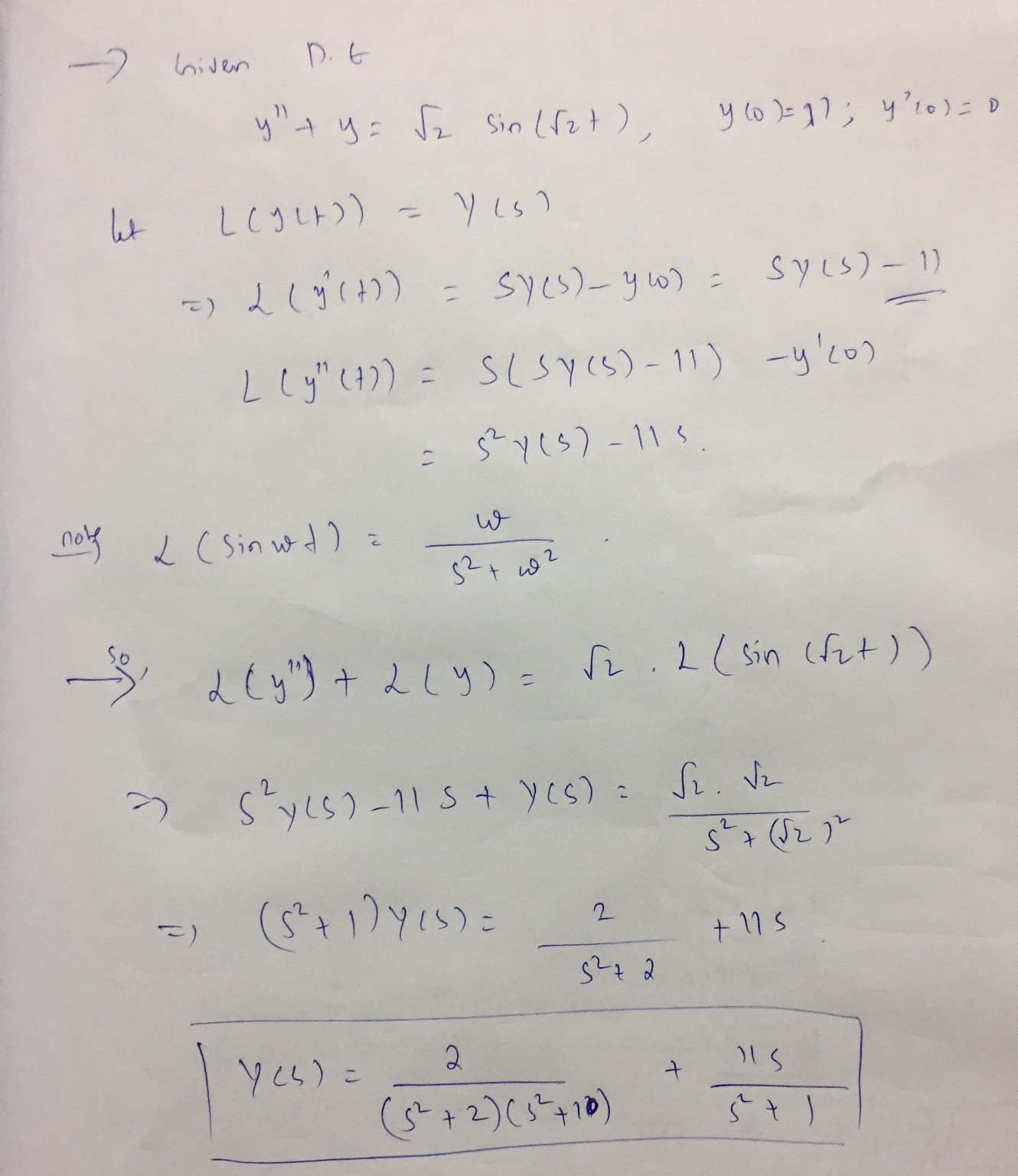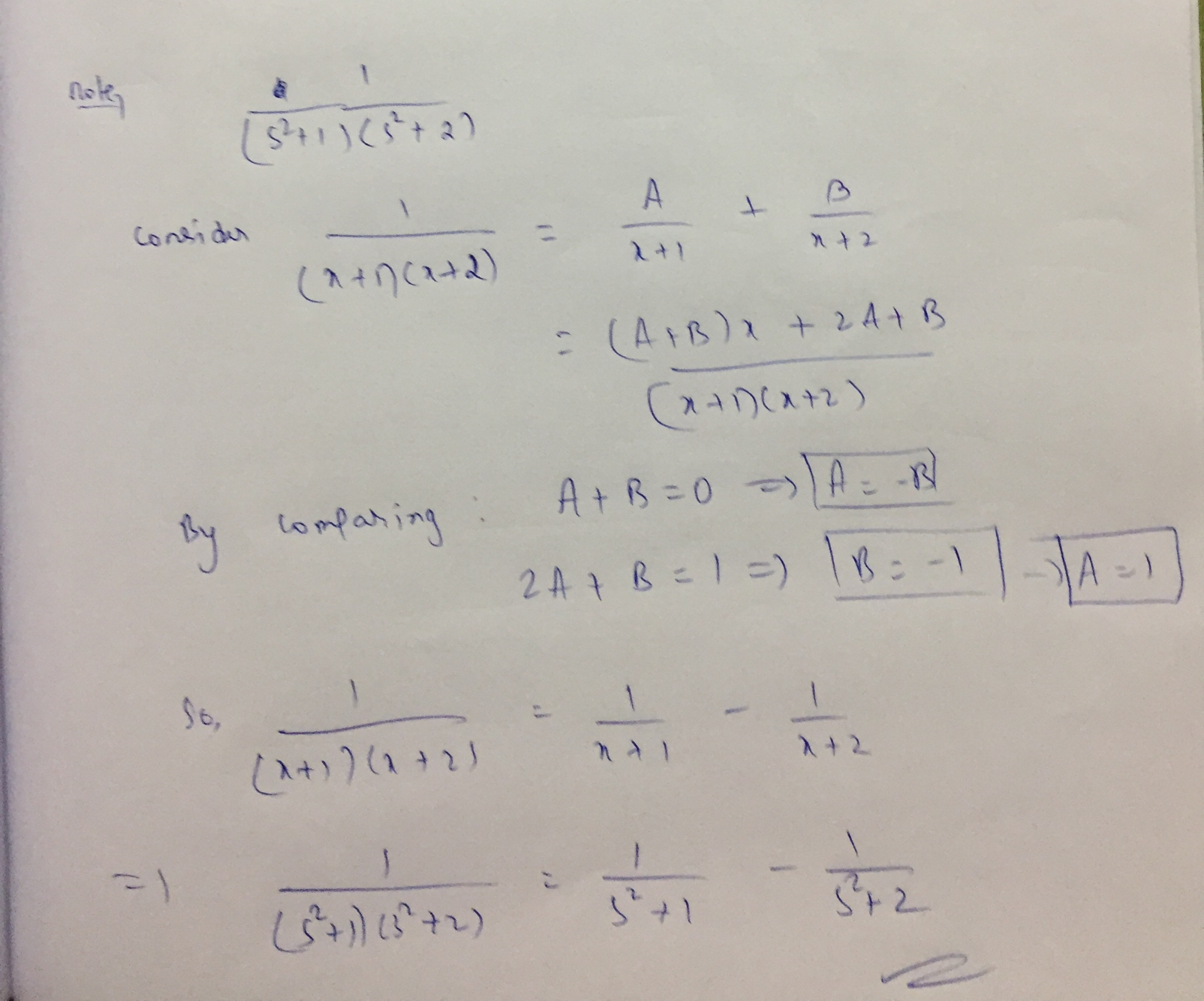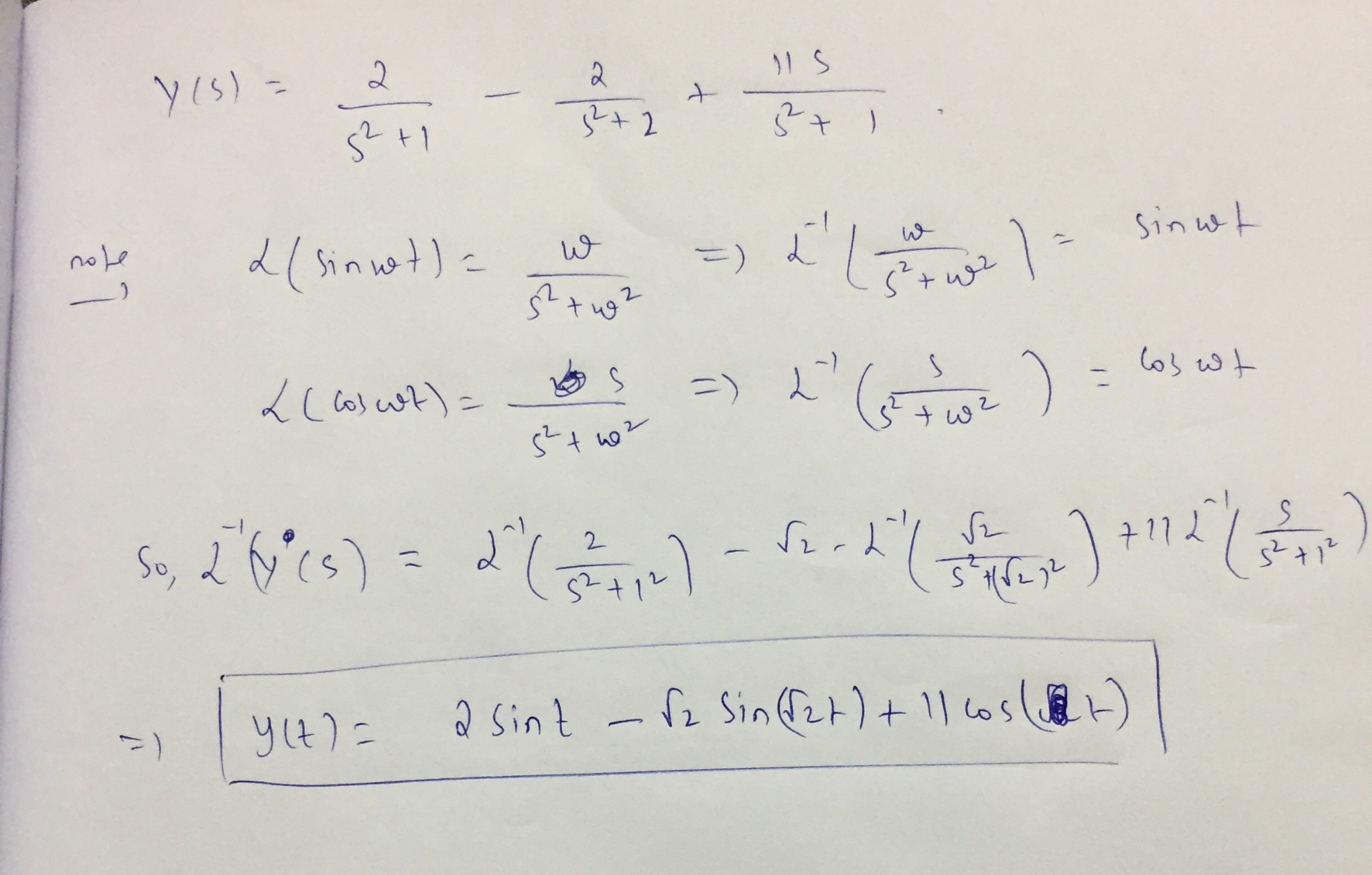Similar Homework Help Questions
• ### 9. DETAILS ZILLDIFFEQMODAP11 7.2.037.MI. MY NOTES ASK YOUR TEACHER Use the Laplace transform to solve the...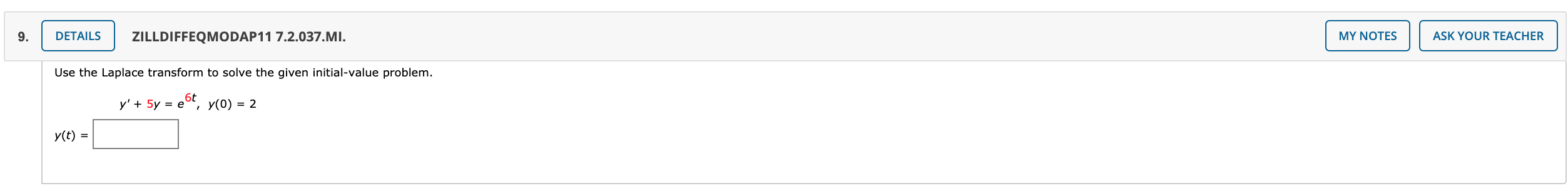9. DETAILS ZILLDIFFEQMODAP11 7.2.037.MI. MY NOTES ASK YOUR TEACHER Use the Laplace transform to solve the given initial-value problem. y' + 5y = e6t, y(0) = 2 y(t) =

• ### 5 -1 points ZillDiffEQModAp11 7.1.015. Ask Your Teacher My Notes Use Definition 7.1.1 Laplace Transform DEFINITION...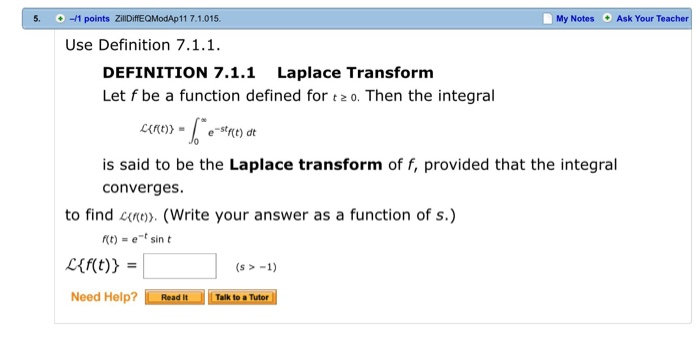5 -1 points ZillDiffEQModAp11 7.1.015. Ask Your Teacher My Notes Use Definition 7.1.1 Laplace Transform DEFINITION 7.1.1 Let f be a function defined fort2 0. Then the integral -stc) d¢ is said to be the Laplace transform of f, provided that the integral converges to find n (Write your answer as a function of s.) f(t)et sin t Lff(t) (s-1) Need Help? Read It Talk to a Tutor

• ### Previous Answers ZillDiffEQ9 7.3.063. Ask Your Teacher 15 1/3 points My Notes Use the Laplace transform...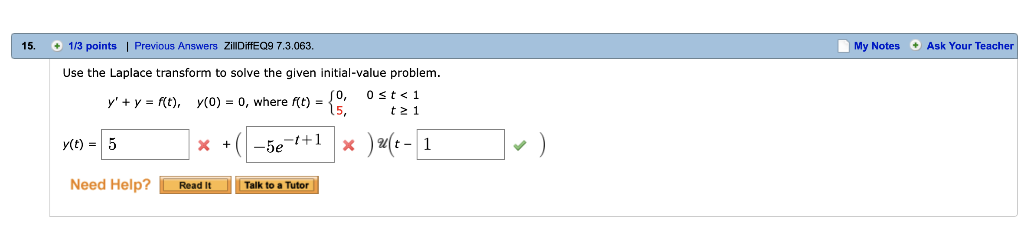Previous Answers ZillDiffEQ9 7.3.063. Ask Your Teacher 15 1/3 points My Notes Use the Laplace transform to solve the given initial-value problem. 0 st<1 y'y f(t), y(0) 0, where f(t) t 2 1 a4c-11 y(t) 5 -5et+1 Need Help? Talk to a Tutor Read It

• ### /2 POINTS ZILLDIFFEQMODAP11 4.4.042 MY NOTES ASK YOUR TEACHER Solve the given initial value problem in...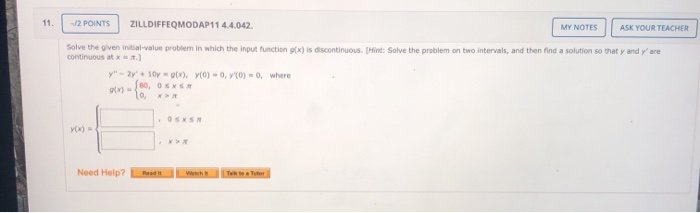/2 POINTS ZILLDIFFEQMODAP11 4.4.042 MY NOTES ASK YOUR TEACHER Solve the given initial value problem in which the input function of) is discontinuous Hint: Solve the problem on two intervals, and then find a solution so that y and continuous at are 0) = 0, where y" - 2y + 10 (x), YO) - 0, ()-80, OSX lo Need Help? Red Wich t e Tutor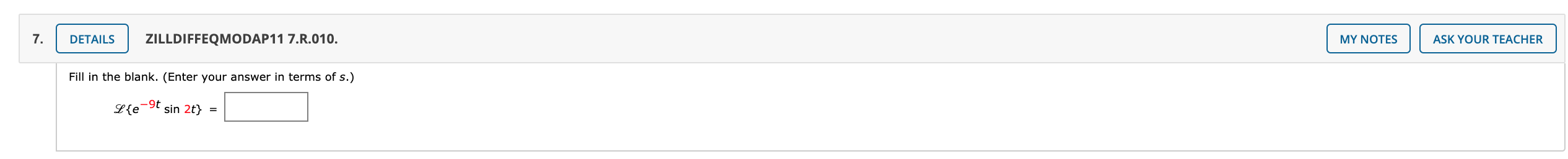7. DETAILS ZILLDIFFEQMODAP11 7.R.010. MY NOTES ASK YOUR TEACHER Fill in the blank. (Enter your answer in terms of s.) L{e -90 sin 2t} =

• ### 4. [-/3 Points] DETAILS ZILLDIFFEQMODAP11 7.5.007.MI. Use the Laplace transform to solve the given initial-value problem....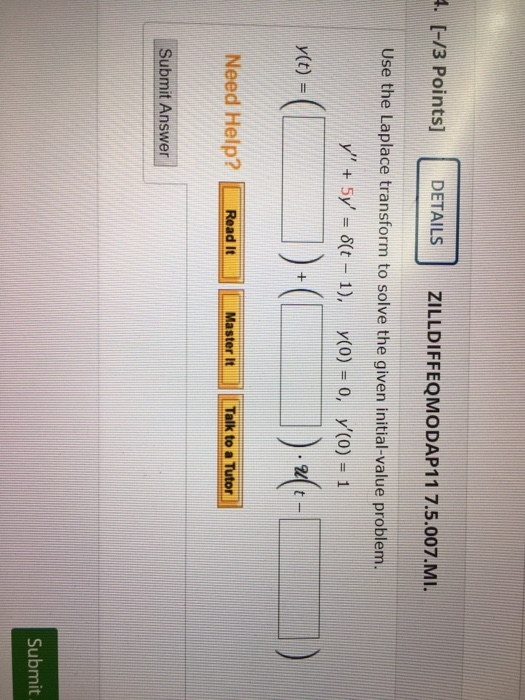4. [-/3 Points] DETAILS ZILLDIFFEQMODAP11 7.5.007.MI. Use the Laplace transform to solve the given initial-value problem. y" + 5y = \$(t - 1), y(0) = 0, 7(0) = 1 y(t) = 1)+(! 1)-2-1 Need Help? Read It Master It Talk to a Tutor Submit Answer Submit

• ### -14 POINTS ZILLDIFFEQMODAP11 7.2.041. Use the Laplace transform to solve the given initial-value pro y"' +...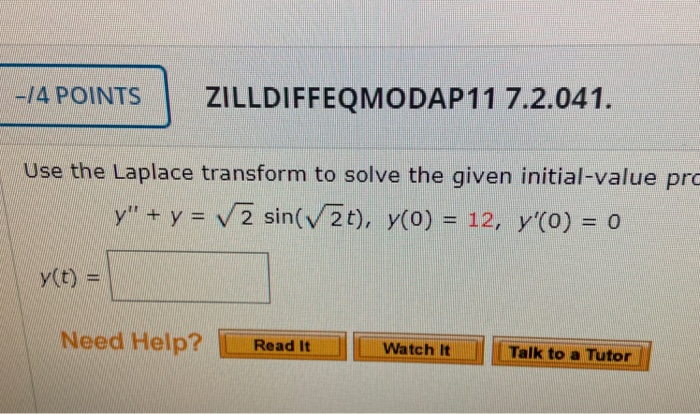-14 POINTS ZILLDIFFEQMODAP11 7.2.041. Use the Laplace transform to solve the given initial-value pro y"' + y = sin(V2t), y(0) = 12, y'(O) = 0 y(t) = Need Help? Read It Watch It Talk to a Tutor

• ### 5. DETAILS ZILLDIFFEQMODAP11 6.R.003. MY NOTES ASK YOUR TEACHER Both power series solutions of y" +...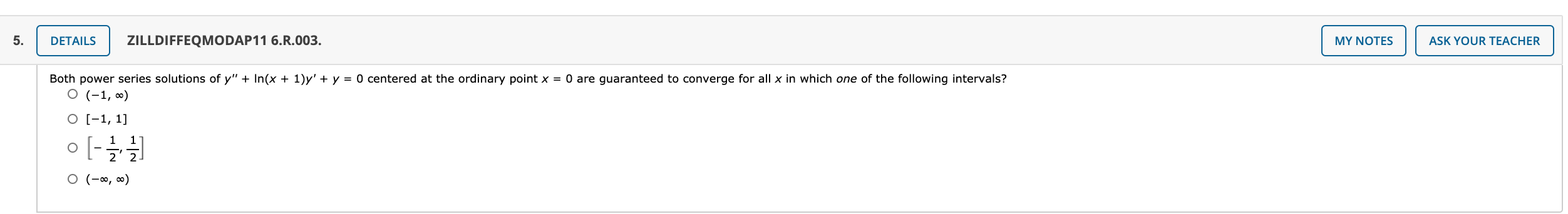5. DETAILS ZILLDIFFEQMODAP11 6.R.003. MY NOTES ASK YOUR TEACHER Both power series solutions of y" + In(x + 1)y' + y = 0 centered at the ordinary point x = 0 are guaranteed to converge for all x in which one of the following intervals? O (-1,0) O [-1, 1] o [-2] O (-0, 0)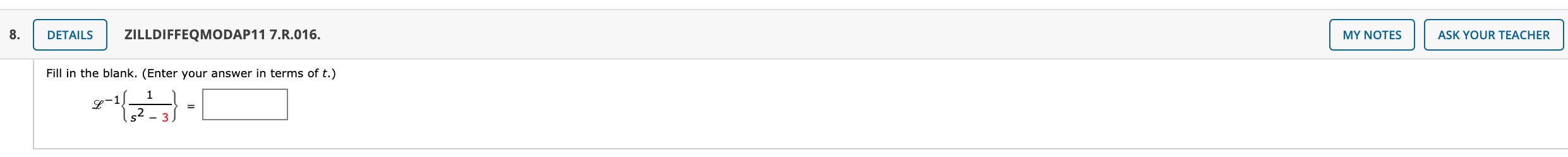8. DETAILS ZILLDIFFEQMODAP11 7.R.016. MY NOTES ASK YOUR TEACHER Fill in the blank. (Enter your answer in terms of t.) 1 S 3

• ### 4. DETAILS ZILLDIFFEQMODAP11 5.R.021. MY NOTES ASK YOUR TEACHER A series circuit contains an inductance of...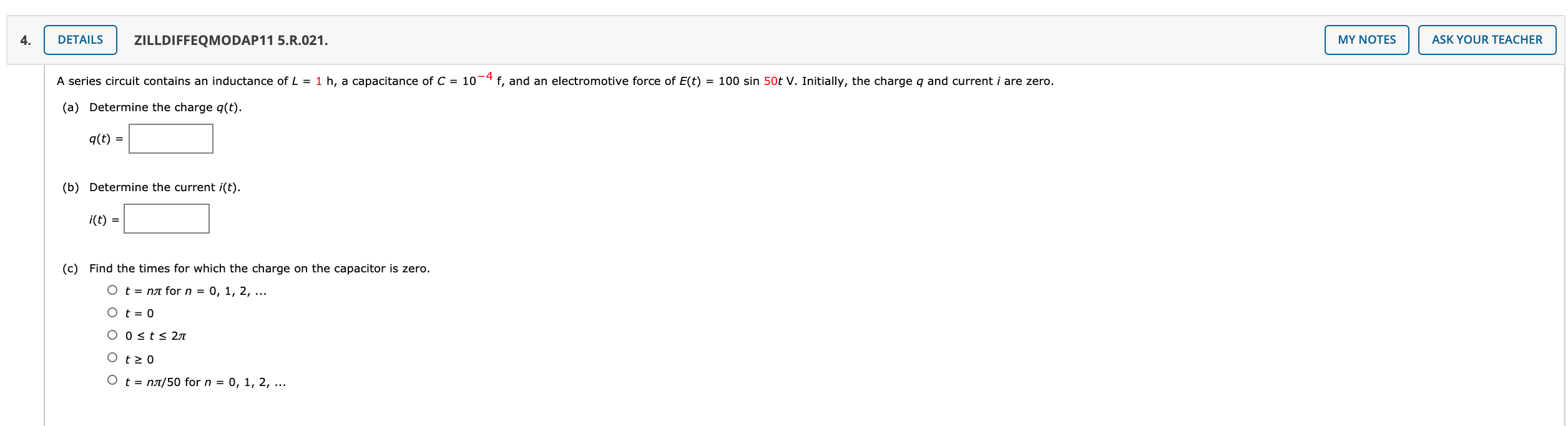4. DETAILS ZILLDIFFEQMODAP11 5.R.021. MY NOTES ASK YOUR TEACHER A series circuit contains an inductance of L = 1 h, a capacitance of C = 10-4 f, and an electromotive force of E(t) = 100 sin 50t V. Initially, the charge q and current i are zero. (a) Determine the charge q(t). q(t) = (b) Determine the current i(t). i(t) = (C) Find the times for which the charge on the capacitor is zero. O t = na for n...

Free Homework App